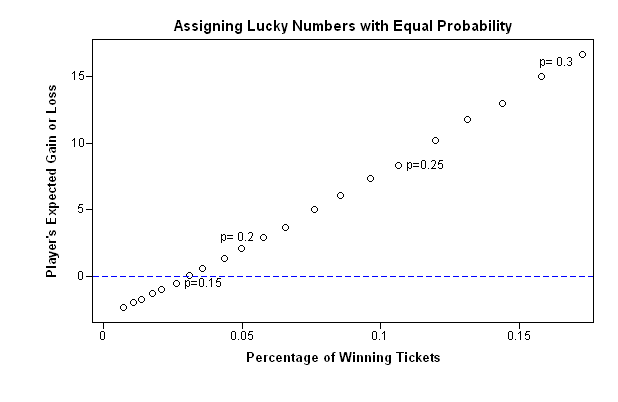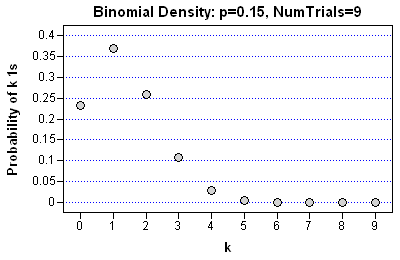Because of this week's story about a geostatistician, Mohan Srivastava, who figured out how predict winning tickets in a scratch-off lottery, I've been thinking about scratch-off games. He discovered how to predict winners when he began to "wonder how they make these [games]."

Each ticket has a set of "lucky numbers." A ticket is a winner if the lucky numbers align on a tic-tac-toe grid along any row, column, or diagonal. The scratch-off portion of the game involves scratching an area on the ticket to reveal the lucky numbers. See my previous blog article for further details.

Srivastava's observation was that the "lucky numbers" on the tic-tac-toe grid have to occur less frequently than the other numbers. Otherwise, the game will pay out more money than it takes in.

### Simplifying the Game for Analysis

How might someone design a tic-tac-toe scratch-off game? I'll simplify the problem by eliminating the lucky numbers, the scratch-off portion of the ticket, and all but one 3x3 grid. Represent all the lucky numbers by a 1, and the remaining numbers by a 0. In this scheme, a card is a winner if it has a row, column, or diagonal of all 1s.

The game costs \$3 to play, and pays out the following amounts:

• If the first row contains all 1s, the payout is \$5.
• If the second row contains all 1s, the payout is \$10.
• If the first row contains all 1s, the payout is \$100.
• If the first column contains all 1s, the payout is \$3 (that is, you get your money back).
• If the second column contains all 1s, the payout is \$20.
• If the third column contains all 1s, the payout is \$100.
• If the either of the diagonals contain all 1s, the payout is \$250.

### What If Lucky Numbers Are Assigned with Equal Probability?

What would happen if the 0s and 1s are assigned to each cell with equal probability? For example, suppose that you specify a 15% chance that any individual cell is assigned a 1. What is the expected gain/loss for that each ticket, and what percentage of tickets will be winners?

The answer is provided by the following simulation and graph. (It is possible to get an exact solution, but I'm feeling lazy.) The simulation constructs a large number of randomly assigned grids. (Each grid is represented by a row of the x matrix.) Each cell of the grid has a certain probability of being a 1. The TestWinner module determines the gain or loss for each grid by determining whether there is a row, column, or diagonal that contains all 1s. The simulation is repeated for a range of probability values, from a 10% probability to a 30% probability.

```proc iml; /** Given N grids (represented by rows of x), determine the payout for each grid. **/ start TestWinner(x); w = j(nrow(x),1, -3); /** gain: initialize to -3 **/ do i = 1 to nrow(x); if all(x[i,1:3]) then w[i] = w[i]+5; if all(x[i,4:6]) then w[i] = w[i]+10; if all(x[i,7:9]) then w[i] = w[i]+100; if all(x[i,{1 4 7}]) then w[i] = w[i]+3; if all(x[i,{2 5 8}]) then w[i] = w[i]+20; if all(x[i,{3 6 9}]) then w[i] = w[i]+100; if all(x[i,{1 5 9}]) then w[i] = w[i]+250; if all(x[i,{3 5 7}]) then w[i] = w[i]+250; end; return(w); finish;   call randseed(54321); NSim = 1E5; x = j(NSim,9); /** each row of x is a grid **/   p = do(0.1, 0.3, 0.01); pctWin = j(ncol(p),1); ExpWin = pctWin; do i = 1 to ncol(p); call randgen(x, "BERNOULLI", p[i]); win = TestWinner(x); ExpWin[i] = win[:]; PctWin[i] = (win>=0)[:]; end;```The graph shows the expected gain or loss when cells in the tic-tac-toe grid are randomly assigned according to a fixed probability value. The graph shows that the lottery corporation (that is, the government) cannot make a profit on games for which the chance of cell being a 1 is more than 15%. However, profitable games that are constructed like this have very few winners. For example, when the chance of a 1 is 15%, only 2.5% of those playing the game get a winning tic-tac-toe combination.

This is a problem for the lottery corporation, because people don't play games that they usually lose. The key to getting people to play a scratch-off game (and slot machines, and other gambling games) is to award many small prizes, but few large prizes. If the 0s and 1s are assigned to each cell with equal probability, then the large cash awards have the same chance of occurring as the smaller awards. The chance of winning has to be set very small (too small!) in order to manage the losses.

This hypothetical analysis shows that it is a bad idea to generate a tic-tac-toe game by using equal probabilities. Instead, cells that contribute to the large cash awards (the diagonals) must be assigned a small probability of getting the "lucky numbers," whereas cells that contribute to small cash awards (the first row and column) can be assigned larger probabilities.

By controlling the probability that each cell contains a lucky number, the lottery corporation can control the chance of winning each prize. It can guarantee a profit for the game, while awarding many small prizes that keep customers coming back to play again.

Srivastava recognized this fact. In the Wired article he is quoted as saying:

It would be really nice if the computer could just spit out random digits. But that's not possible, since the lottery corporation needs to control the number of winning tickets.

### Distribution of the Lucky Numbers

As a statistical footnote to this analysis, you can construct the frequency distribution of the number of 1s in a tic-tac-toe grid that uses a random uniform assignment for each cell. The count of 1s follows a binomial distribution. For example, if the probability that a cell contains a 1 is 15%, the following SAS/IML statements compute the frequency distribution of 1s in the grid:

```/** distribution of 1s in a 3x3 grid in which each cell has a 15% chance of a 1 **/ k = 0:9; pdf = pdf("Binomial", k, 0.15, 9);```The scatter plot shows the probability that a tic-tac-toe grid will have a given number, k, of 1s for k=0, 1, 2,...,9, when there is a 15% chance that a given cell contains a 1. The graph shows that most grids (86%) that are constructed under this scheme will have zero, one, or two 1s. Obviously, none of these grids can be a winning ticket.

Once again, the conclusion is that a game that is constructed using this scheme would not be fun to play: the "lucky numbers" don't appear often enough! In order to increase the frequency of lucky numbers while managing the risk of the tickets that pay out, you need to use a construction method that assigns different probabilities to each cell of the grid.

I'll describe that approach tomorrow.

Share